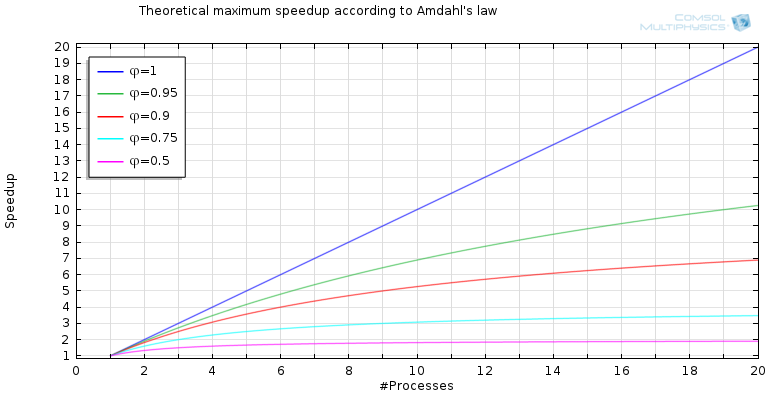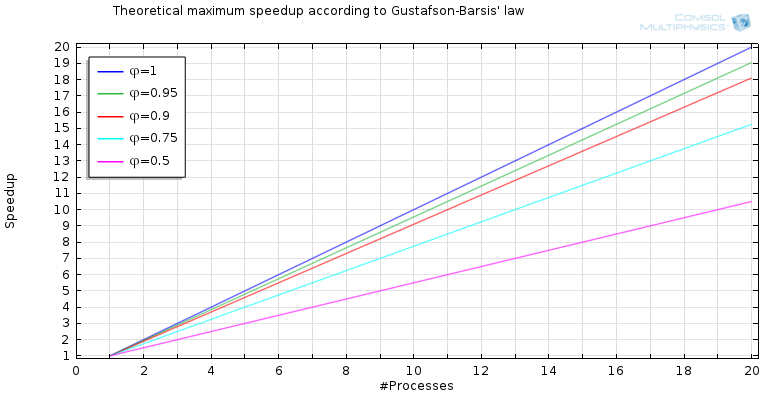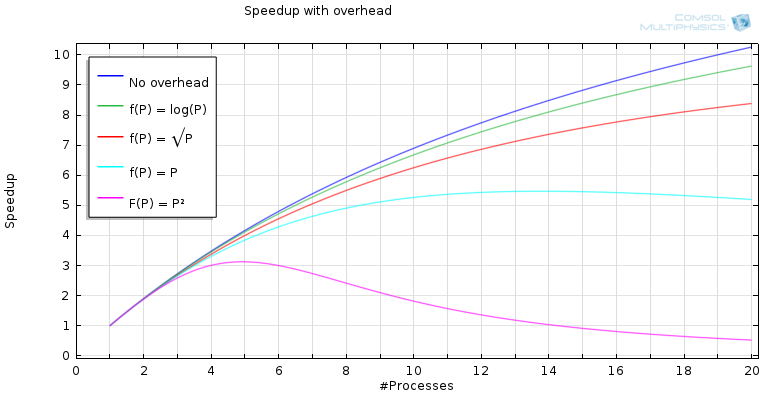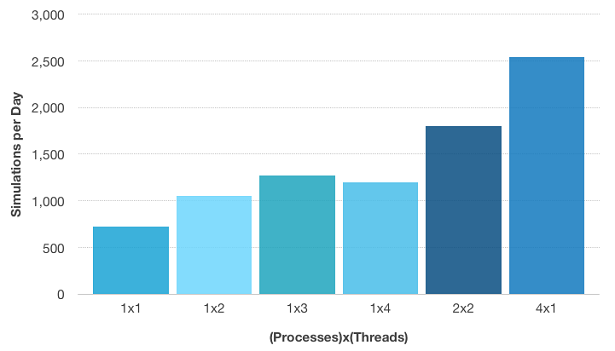#####Pär Persson Mattsson March 20, 2014

One thing we haven’t talked much about so far in the Hybrid Modeling blog series is what speedup we can expect when adding more resources to our computations. Today, we consider some theoretical investigations that explain the limitations in parallel computing. We will also show you how to use the COMSOL software’s Batch Sweeps option, which is a built-in, embarrassingly parallel functionality for improving performance when you reach these limits.

### Amdahl’s and Gustafson-Barsis’ laws

We have mentioned before how speedup through the addition of compute units is dependent on the algorithm (in this post we will use the term processes, but added compute units can also be threads). A strictly serial algorithm, like computing the elements of the Fibonacci series, does not benefit at all from an added process, while a parallel algorithm, like vector addition, can make use of as many processors as we have elements in the vector. Most algorithms in the real world are somewhere in between these two.

To analyze the possible maximum speedup of an algorithm, we will assume that it consists of a fraction of perfectly parallelizable code and a fraction of strictly serial code. Let us call the fraction of parallelized code \varphi, where \varphi is a number between (and including) 0 and 1. This automatically means that our algorithm has a fraction of serial code that is equal to (1-\varphi).

Considering the computation time, T(P), for P active processes, and starting with the case P=1, we can use the representation T(1) = T(1) \cdot(\varphi + (1-\varphi)). When running P processes, the serial fraction of the code is not affected, but the perfectly parallelized code will be computed P times faster. Therefore, the computation time for P processes is T(P)=T(1) \cdot (\varphi / P + (1 -\varphi)), and the speedup is S(P):=T(1)/T(P)=1/(\varphi/P+(1-\varphi)).

#### Amdahl’s Law

This expression is at the heart of Amdahl’s law. Plotting S(P) for different values of \varphi and P, we now see something interesting in the graph below.The speedup for increasing the number of processes for different fractions of parallelizable code.

For 100% parallelized code, the sky is the limit. Yet, we find that for \varphi<1, the asymptotic limit or the theoretical maximal speedup is S_{max}(\varphi):=\lim_{P\to \infty} S(P)=1/(1-\varphi).

For a 95% parallelized code, we find that S_{max}(0.95)=20 — a maximum speedup of twenty times, even if we have an infinite number of processes. Furthermore, we have S_{max}(0.9)=10, S_{max}(0.75)=4, and S_{max}(0.5)=2. The theoretical maximum speedup decreases quickly when decreasing the fraction of parallelized code.

But don’t give up and go home just yet!

#### Gustafson-Barsis’ Law

There is one thing that Amdahl’s law does not consider, and that is the fact that when we buy a faster and larger computer to be able to run more processes, we usually don’t want to compute our small models from yesterday faster. Instead, we want to compute new, larger (and cooler) models. That’s what the Gustafson-Barsis’ law is all about. This is based on the assumption that the size of the problem we want to compute increases linearly with the number of available processes.

Amdahl’s law assumes that the size of the problem is fixed. When adding new processors they are working on parts of the problem that was originally handled by a lesser number of processes. By adding more and more processes, you are not utilizing the full ability of the added processes as eventually the size of what they are able to work on reaches a lower limit. Yet, by assuming that the size of the problem increases with the number of added processes, then you are utilizing all the processes to an assumed level, and the speedup of the performed computations remains unbounded.

The equation describing this phenomenon is S(P)=\phi\cdot P-(1-\phi), which gives us a far more optimistic result for what is called scaled speedup (which is like productivity), as shown in the graph below:When taking into account that the size of the job normally increases with the number of available processes, our predictions are more optimistic.

### The Cost of Communication

Gustafson-Barsis’ law implies that we are only restricted in the size of the problem we can compute by the resources we have for adding processes. Yet, there are other factors that affect speedup. Something we’ve tried to stress so far in this blog series is that communication is expensive. But we haven’t talked about how expensive it is yet, so let’s look at some examples.

Let’s consider an overhead that is dominated by the communication and synchronization required in parallel processing, and model this as time added to the computation time. This means that the amount of communication increases when we increase the number of processes, and that this increase will be modeled by a function OH(P)=c\cdot f(P), where c is a constant and f(P) is some function. Hence, we can compute the speedup through: S(P)_{OH}=1/(\varphi/P+(1-\varphi)+c \cdot f(P)).

The graph below shows the case where the fraction of parallelized code is 95%, and where we can see the speedup for an increasing number of processes, for different functions of f(P), assuming c= 0.005 (this constant would vary between different problems and platforms). In the case of no overhead, the result is as predicted by Amdahl’s law, but when we start adding overhead, we see that something is happening.

For a linearly increasing overhead, we find that the speedup doesn’t reach a value greater than five before the communication starts to counteract the increased computation power added by more processes. For a quadratic function, f(P), the result is even worse and, as you might recall from our earlier blog post on distributed memory computing, the increase of communication is quadratic in the case of all-to-all communication.Speedup with added overhead. The constant, c, is chosen to be 0.005.

Due to this phenomenon, we cannot expect to have a speedup on a cluster for, say, a small time-dependent problem when adding more and more processes. The amount of communication would increase faster than any gain from added processes. Yet, in this case, we have only considered a fixed problem size and the “slowdown” effect introduced through communication would be less relevant as we increase the size of our problem.

### Batch Sweeps in COMSOL Multiphysics

Let us now leave the theoretical aspect and learn how to make use of the batch sweep feature in COMSOL Multiphysics. As our example model, we will use the electrodeless lamp, which is available in the Model Gallery. This model is small, at around 80,000 degrees of freedom, but needs about 130 time steps in its solution. To make this transient model parametric as well, we will compute the model for several values of the lamp power, namely 50 W, 60 W, 70 W, and 80 W.

On my workstation, a Fujitsu® CELSIUS® equipped with an Intel® Xeon® E5-2643 quad core processor and 16 GB of RAM, the following compute times are received:

Number of Cores Compute Time per Parameter Compute Time for Sweep
1 30 mins 120 mins
2 21 mins 82 mins
3 17 mins 68 mins
4 18 mins 72 mins

The speedup here is far from perfect — just about 1.7 for three cores and has even decreased for four cores. This is due to fact that it is a small model with a low number of degrees of freedom per thread within each time step.

We will now use the batch sweep functionality to parallelize this problem in another way: we will switch from data parallelism to task parallelism. We will create a batch job for each parameter value and see what this does to our computation times. To do this, we first activate the “Advanced Study Options”, then we right-click on “Study 1” and choose “Batch Sweep”, as illustrated in the animation below:

How to activate batch sweep in a model, include the parameter values, and specify the number of simultaneous jobs.

The graph below indicates the productivity or “speedup” we can get by controlling the parallelization. When running one batch job using four cores, we get the result from above: 72 minutes. When changing the configuration to two batch jobs simultaneously, each using two cores, we can compute all the parameters in 48 minutes. Finally, when computing four batch jobs at the same time, each using one processor, the total computation time is 34 minutes. This gives speedups of 2.5 and 3.5 times, respectively — a lot better compared to parallelizing through using pure shared memory alone.Simulations per day for the electrodeless lamp model. “4×1” means four batch jobs run simultaneously, using one core each.

### Concluding the Hybrid Modeling Series

Throughout this blog series, we have learned about shared, distributed, and hybrid memory computing and what their weaknesses and strengths are, as well as the large potential of parallel computing. We have also learned that there is no such thing as a free lunch when it comes to computing; we cannot just add processes and hope for perfect speedup for all types of problems.

Instead, we need to choose the best way to parallelize a problem to get the most performance gain out of our hardware, much like we have to choose the correct solver to get the best solution time when solving a numerical problem.

Selecting the right parallel configuration is not always easy, and it can be hard to know beforehand how you should “hybridize” your parallel computations. But as in many other cases, experience comes from playing around and testing, and with COMSOL Multiphysics, you have the possibility to do that. Try it yourself with different configurations and different models, and you will soon know how to set the software up in order to get the best performance out of your hardware.

Fujitsu is a registered trademark of Fujitsu Limited in the United States and other countries. CELSIUS is a registered trademark of Fujitsu Technology Solutions in the United States and other countries. Intel and Xeon are trademarks of Intel Corporation in the U.S. and/or other countries.

#### Post Tags

Clusters Hybrid Modeling series

Calculate the Force of a One-Sided Magnet